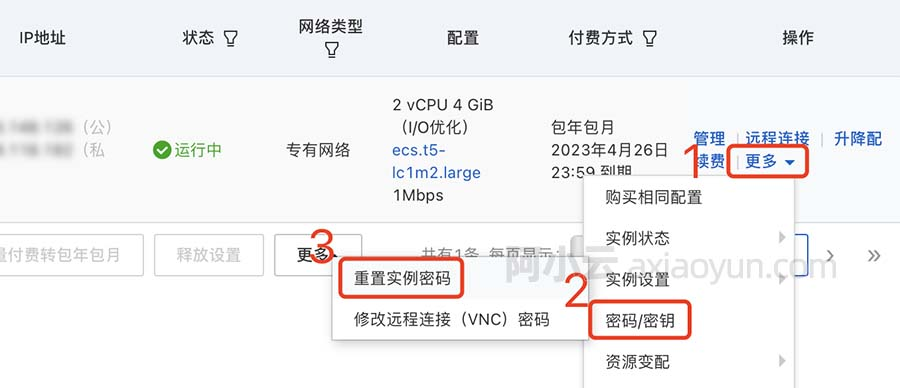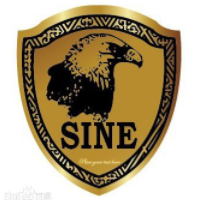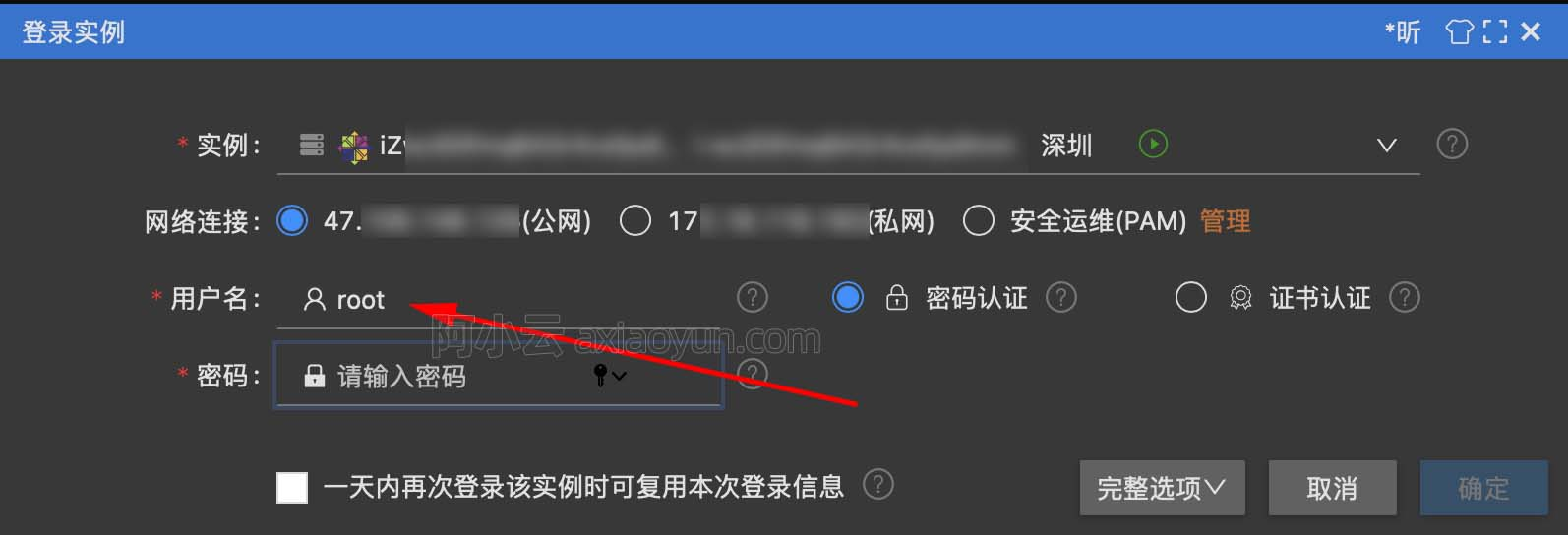【PTA】7-3 判断上三角矩阵 (15分)

+关注继续查看

2
3
1 2 3
0 4 5
0 0 6
2
1 0
-8 2

YES
NO

#include <stdio.h>
int f(int n){
int sum=0;
while(n>0){
sum+=n;
n--;
}
return sum;
}
int main()
{
int T;
scanf("%d",&T);
int n;
for(int i=0;i<T;i++){
scanf("%d",&n);
int arr[n][n],count=0;
for(int j=0;j<n;j++){
for(int k=0;k<n;k++){
scanf("%d",&arr[j][k]);
if(j>k&&arr[j][k]==0) count++;
}
}
if(count==f(n-1)) printf("YES");
else printf("NO");
if(i!=T-1) printf("\n");
}
return 0;
}8102 026170 010513 09879 0windows server 2008阿里云ECS服务器安全设置

8386 011638 06913 053296 03252 021238 0+关注
35

0

《2021云上架构与运维峰会演讲合集》

《零基础CSS入门教程》

《零基础HTML入门教程》